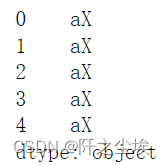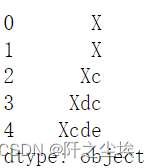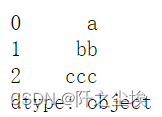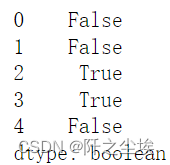﻿ Pandas数据分析之pandas文本处理_python_脚本之家
python# Pandas数据分析之pandas文本处理

## 前言

pandas对文本数据也有很多便捷处理方法，可以不用写循环，向量化操作运算速度快，还可以进行高级的正则表达式，各种复杂的逻辑筛选和匹配提取信息。对于机器学习来说，从文本中做特征工程很是很有用的。

```import numpy as np
import pandas as pd
data = 'https://www.gairuo.com/file/data/dataset/team.xlsx'

## 文本数据类型

#object 和 StringDtype 是 Pandas 的两个文本类型，不过作为新的数据类型，官方推荐 StringDtype 的使用。
#默认情况下，文本数据会被推断为 object 类型。

`pd.Series(['a', 'b', 'c'])`string 类型需要专门进行指定：

```pd.Series(['a', 'b', 'c'], dtype="string")
pd.Series(['a', 'b', 'c'], dtype=pd.StringDtype())```​转换,可以从其他类型转换到这两个类型：

```s = pd.Series(['a', 'b', 'c'])
s.astype("object") # 转换为 object
s.astype("string") # 转换为 string
# 类型转换，支持 string 类型
df.convert_dtypes().dtypes```

## 字符操作

```s = pd.Series(['A', 'B', 'C', 'Aaba','Baca', np.nan, 'CABA','dog', 'cat'], dtype="string")
s````s.str.lower()# 转为小写````# 转为 object
df.Q1.astype(str).str
# 转为 StringDtype
df.team.astype("string").str
df.Q1.astype(str).astype("string").str```​.str后要展示数据要进行分割

```#.str后要展示数据要进行分割
df.team.astype("string").str.strip()#等价于df.team.astype("string")```对索引进行操作：

```df.index.str.lower()
# 对表头，列名进行操作
df.columns.str.lower()
df.columns.str.strip()     #相当于df.columns
#如果对数据连续进行字符操作，则每个操作都要使用 .str 方法：
df.columns.str.strip().str.lower().str.replace('q', '_')```## 文本格式

```#格式转换
s = pd.Series(['lower', 'CAPITALS', 'this is a sentence', 'SwApCaSe'])
s.str.lower() # 转为小写
s.str.upper() # 转为大写
s.str.title() # 标题格式，每个单词大写
s.str.capitalize() # 首字母大写
s.str.swapcase() # 大小写互换
s.str.casefold() # 转为小写，支持其他语言如德语```### 文本对齐

```# 居中对齐，宽度为10，用 - 填充
s.str.center(10, fillchar='-')
# 左对齐
s.str.ljust(10, fillchar='-')
# 右对齐
s.str.rjust(10, fillchar='-')```​指定宽度，填充内容对齐方式，填充内容

```# side{‘left', ‘right', ‘both'}, default ‘left'
# 填充对齐
s.str.zfill(10) # 生成字符，不足10位的前边加0```### ​文本计数和编码

```# 指定字母的数量
s.str.count('a')
# 支持正则，包含 abc 三个字母的总数
s.str.count(r'a|b|c')
# 字符长度
s.str.len()``````# 编码
s.str.encode('utf-8')
# 解码
s.str.decode('utf-8')
# 字符串的Unicode普通格式
# form{‘NFC', ‘NFKC', ‘NFD', ‘NFKD'}
s.str.normalize('NFC')```### 格式判断

#类别判断,以下方法可以判断文本的相关格式：

```# 检查字母和数字字符
s.str.isalpha() # 是否纯英文数字单词组成
s.str.isalnum() # 是否单词、数字或者它们组合形式组成```请注意，对于字母数字检查，针对混合了任何额外标点或空格的字符的检查将计算为 False

```s.str.isdecimal() # 是否数字 0-9 组成合规10进制数字
s.str.isdigit() # 同 但可识别 unicode中的上标和下标数字
s.str.isnumeric() # 是否可识别为一个数字，同 isdigit 可识别分数
s.str.isdecimal()  #是否为小数

s.str.isspace() # 是否空格
s.str.islower() # 是否小写
s.str.isupper() # 是否大写
s.str.istitle() # 是否标题格式，只有第一个字母大写```wrap将长文本拆分开指定宽度的字符，用换行连接

`s.str.wrap(10) `## 文本高级处理

### 文本分割

```#对内容中的下划线进行了分隔，分隔后每个内容都成为了一个列表，其中对空值是不起作用的。
s2 = pd.Series(['a_b_c', 'c_d_e', np.nan, 'f_g_h'], dtype="string")
s2.str.split('_')````s2.str.split('_').str````# get 只能传一个值
s2.str.split('_').str.get(1)``````# [] 可以使用切片操作
s2.str.split('_').str[1:3]
s2.str.split('_').str[:-2]
# 如果不指定分隔符，会按空格进行分隔
s2.str.split()
# 限制分隔的次数，从左开始，剩余的不分隔
s2.str.split(n=2)```

##字符展开,使用 split 可以将分隔后的数据展开形成新的行内容。

`s2.str.split('_', expand=True)`# 指定展开列数，n 为切片右值

`s2.str.split('_', expand=True, n=1)`#rsplit 和 split一样，只不过它是从右边开始分隔，如果没有n参数，rsplit和split的输出是相同的。

`s2.str.rsplit('_', expand=True, n=1)`#使用正则，对于规则比较复杂的，分隔符处可以传入正则表达式：

```s = pd.Series(["1+1=2"])
s.str.split(r"\+|=", expand=True)```### 文本切片选择 slice

#可以使用 .str.slice() 将指定的内容切除掉，不过还是推荐使用 s.str[]来实现，这样和Python字符串列表操作是一样的

```s = pd.Series(["koala", "fox", "chameleon"])
s.str.slice() # 不做任何事
s.str.slice(1) # 切掉第一个字符
s.str.slice(start=1) #同上```其他参数用法：

```# 切除最后一个以前的，留下最后一个
s.str.slice(start=-1) # s.str[-1]
# 切除第二位以后的
s.str.slice(stop=2) # s.str[:2]
# 切除步长为2的内容
s.str.slice(step=2) # s.str[::2]
# 切除从开头开始，第4位以后并且步长在3的内容
# 同 s.str[0:5:3]
s.str.slice(start=0, stop=5, step=3)```

### 划分 partition

#.str.partition可以将文本按分隔符号划分为三个部分，形成一个新的 DataFrame或者相关数据类型。

```s = pd.Series(['Linda van der Berg', 'George Pitt-Rivers'])
s.str.partition()```其他：

```# 从右开始划分
s.str.rpartition()
# 指定符号
s.str.partition('-')
# 划分为一个元组列
s.str.partition('-', expand=False)
# 对索引进行划分
idx = pd.Index(['X 123', 'Y 999'])
idx.str.rpartition()      ```### 文本替换

```s = pd.Series(['12', '-\$10', '\$10,000'], dtype="string")
s.str.replace('\$', '')````s.str.replace(r'\$|,', '')#逗号也替换`#如果我们替换 -\$ 则发现不起作用，是因为替换字符默认是支持正则的（可以使用 regex=False 不支持），可以进行转义来实现。

```s.str.replace('-\$', '') # 不起作用
s.str.replace(r'-\\$', '-') # 进行转义后正常```

### 指定位置替换

#slice_replace 可以将保留选定内容，剩余内容进行替换：

```s = pd.Series(['a', 'ab', 'abc', 'abdc', 'abcde'])
# 保留第一个，其他的替换或者追加 X
s.str.slice_replace(1, repl='X')```# 指定位置前删除并用 X 替换

`s.str.slice_replace(stop=2, repl='X')`# 指定区间的内容被替换

`s.str.slice_replace(start=1, stop=3, repl='X')`### 重复替换

```# 对整体重复两次
pd.Series(['a', 'b', 'c']).repeat(repeats=2)
# 对每个行内的内容重复两次
pd.Series(['a', 'b', 'c']).str.repeat(repeats=2)
# 指定每行重复几次
pd.Series(['a', 'b', 'c']).str.repeat(repeats=[1, 2, 3])```### 文本连接

#方法 s.str.cat 可以做文本连接的功能，下面介绍如何将序列的文本或者两个文本序列连接在一起的方法。

```#自身的连接
s = pd.Series(['a', 'b', 'c', 'd'], dtype="string")
s.str.cat(sep=',')
# 'a,b,c,d'
s.str.cat()```对空值的处理：

```t = pd.Series(['a', 'b', np.nan, 'd'], dtype="string")
t.str.cat(sep=',')
#'a,b,d'
t.str.cat(sep=',', na_rep='-')```#指定列表序列连接

`s.str.cat(['A', 'B', 'C', 'D'])``s.str.cat(t, na_rep='-')#空值处理`当然我们也可以使用 pd.concat 来进行链接两个序列:

```d = pd.concat([t, s], axis=1)
'''
0	1
0	a	a
1	b	b
2	<NA>	c
3	d	d'''
# 两次连接
s.str.cat(d, na_rep='-')``````u = pd.Series(['b', 'd', 'a', 'c'],
index=[1, 3, 0, 2],
dtype="string")
# 以左边索的为主
s.str.cat(u)
s.str.cat(u, join='left')
# 以右边的索引为主
s.str.cat(u, join='right')
# 其他
s.str.cat(t, join='outer', na_rep='-')
s.str.cat(t, join='inner', na_rep='-')```

### 文本查询

#查询 findall

```s = pd.Series(['Lion', 'Monkey', 'Rabbit'])
s.str.findall('Monkey')
'''
0          []
1    [Monkey]
2          []
dtype: object
'''
# 大小写敏感，不会查出内容
s.str.findall('MONKEY')````s.str.findall('on')   #包含on`#利用正则查询和给定文本相同的内容：

```# 忽略大小写
import re
s.str.findall('MONKEY', flags=re.IGNORECASE)
# 以 on 结尾
s.str.findall('on\$')
# 包含多个的会形成一个列表
s.str.findall('b')```#可以使用str.find匹配返回匹配结果的位置（从0开始），-1为不匹配：

`s.str.find('Monkey')````s1 = pd.Series(['Mouse', 'dog', 'house and parrot', '23', np.NaN])
s1.str.contains('og', regex=False)```### 文本包含

#包含 contains
#判断字符是否有包含关系，经常用在数据筛选中。它默认是支持正则的，如果不需要可以关掉。na=nan 可以指定

```s1 = pd.Series(['Mouse', 'dog', 'house and parrot', '23', np.NaN])
s1.str.contains('og', regex=False)```#可以用在数据查询筛选中：

```# 名字包含 A 字母
df.loc[df.name.str.contains('A')]
# 包含 A 或者 C
df.loc[df.name.str.contains('A|C')]
# 忽略大小写
import re
df.loc[df.name.str.contains('A|C', flags=re.IGNORECASE)]
# 包含数字
df.loc[df.name.str.contains('\d')]```

另外，.str.startswith 和 .str.endswith 还可以指定开头还是结尾包含：

```s = pd.Series(['bat', 'Bear', 'cat', np.nan])
s.str.startswith('b')
# 对空值的处理
s.str.startswith('b', na=False)
s.str.endswith('t')
s.str.endswith('t', na=False)```### 文本匹配

```#匹配 match，确定每个字符串是否与正则表达式匹配。
pd.Series(['1', '2', '3a', '3b', '03c'], dtype="string").str.match(r'[0-9][a-z]')```### 文本提取

#提取 extract， .str.extract 可以利用正则将文本中的数据提取出来形成单独的列，下列中正则将文本分为两部分，
#第一部分匹配 ab 三个字母，第二位匹配数字，最终得这两列，c3 由于无法匹配，最终得到两列空值。

```s=pd.Series(['a1', 'b2', 'c3'],dtype="string")
s.str .extract(r'([ab])(\d)', expand=True)```#expand 参数如果为真则返回一个 DataFrame，不管是一列还是多列，为假时只有一列时才会返回一个 Series/Index。

`s.str.extract(r'([ab])?(\d)')`#3是数值，匹配上了

```# 取正则组的命名为列名
s.str.extract(r'(?P<letter>[ab])(?P<digit>\d)')```#匹配全部，会将一个文本中所有符合规则的匹配出来，最终形成一个多层索引数据：

```s = pd.Series(["a1a2", "b1b7", "c1"],index=["A", "B", "C"], dtype="string")
two_groups = '(?P<letter>[a-z])(?P<digit>[0-9])'
s.str.extract(two_groups, expand=True) # 单次匹配````s.str.extractall(two_groups)`### 提取虚拟变量

#可以从字符串列中提取虚拟变量。 例如用“ |”分隔：

```s = pd.Series(['a', 'a|b', np.nan, 'a|c'], dtype="string")
s.str.get_dummies(sep='|')```#也可以对索引进行这种操作：

```idx = pd.Index(['a', 'a|b', np.nan, 'a|c'])
idx.str.get_dummies(sep='|')```